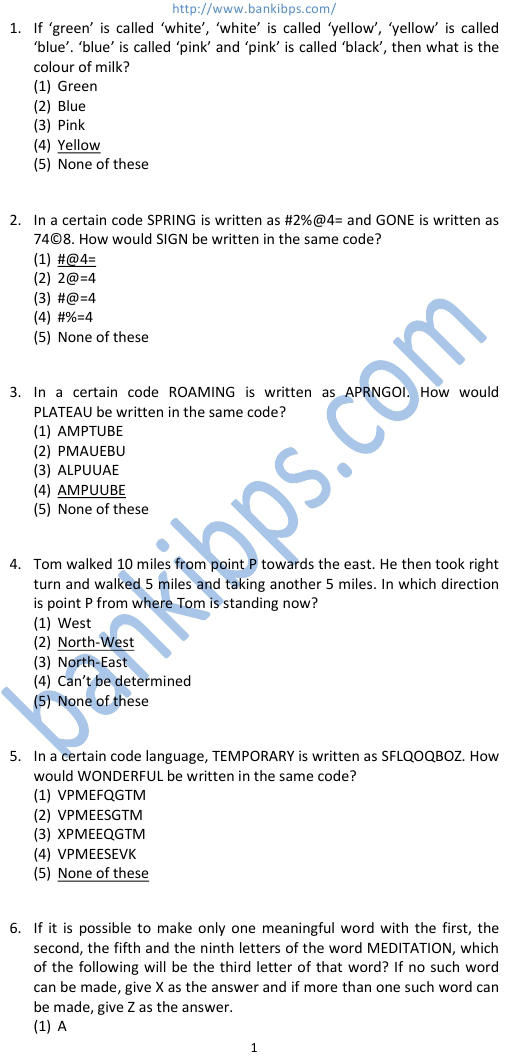# Model Question Paper for LIC Agent Exam

1. If ‘green’ is called ‘white’, ‘white’ is called ‘yellow’, ‘yellow’ is called ‘blue’. ‘blue’ is called ‘pink’ and ‘pink’ is called ‘black’, then what is the colour of milk? (1) Green (2) Blue (3) Pink (4) Yellow (5) None of these model question paper for lic agent exam 2. In a certain code SPRING is written as #2%@4= and GONE is written as 74©8. How would SIGN be written in the same code? (1) #@4= (2) 2@=4 (3) #@=4 (4) #%=4 (5) None of these 3. In a certain code ROAMING is written as APRNGOI. How would PLATEAU be written in the same code? (1) AMPTUBE (2) PMAUEBU (3) ALPUUAE (4) AMPUUBE (5) None of these 4. Tom walked 10 miles from point P towards the east. He then took right turn and walked 5 miles and taking another 5 miles. In which direction is point P from where Tom is standing now? (1) West (2) North-West (3) North-East (4) Can’t be determined (5) None of these
Practice Exercise - 322 [ Reasoning ]## model question paper for lic agent exam

### sample paper for lic agent exam

#### lic agent sample question paper

##### lic sample question papers
###### lic exam sample question paper
sample paper for lic agent exam. lic agent sample question paper. lic sample question papers. lic exam sample question paper. irda exam sample paper. irda model question papers with answers. lic agent exam question papers with answers. irda question paper with answer 2012. lic aao model question papers with answers. irda exam question paper and answers. lic aao question paper with answers. lic exam questions and answers.